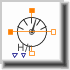Log Frequency Sweep - MapleSim Help

Log Frequency Sweep

Logarithmic frequency sweepDescription The Log Frequency Sweep component generates a logarithmic frequency sweep from ${\mathrm{\omega }}_{\mathrm{min}}$ to ${\mathrm{\omega }}_{\mathrm{max}}$, and then stops.Equations $y={10}^{\mathrm{log10}\left({\mathrm{\omega }}_{\mathrm{min}}\right)+\left(\left(\mathrm{log10}\left({\mathrm{\omega }}_{\mathrm{max}}\right)-\mathrm{log10}\left({\mathrm{\omega }}_{\mathrm{min}}\right)\right)\right)\mathrm{min}\left(1,\frac{t}{\mathrm{max}\left(\mathrm{duration},\mathrm{\epsilon }\right)}\right)}$ $\mathrm{\epsilon }=\mathrm{Modelica.Constants.eps}$Connections

 Name Description Modelica ID $y$ Connector of Real output signal yParameters

 Name Default Units Description Modelica ID ${\mathrm{\omega }}_{\mathrm{min}}$ Lower frequency border wMin ${\mathrm{\omega }}_{\mathrm{max}}$ Upper frequency border wMax start time 0 $s$ Start time of frequency sweep startTime $\mathrm{duration}$ $s$ Duration of ramp durationModelica Standard Library The component described in this topic is from the Modelica Standard Library. To view the original documentation, which includes author and copyright information, click here.